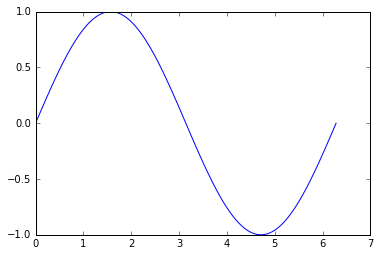Prev: -       |       Next: Basic plotting with 'plot'

What is matplotlib?¶

matplotlib is a python 2D plotting library which produces publication quality figures in a variety of hardcopy formats and interactive environments across platforms

• Thus, matplotlib is the first -and possibly the only- plotting package you will ever need to develop high quality scientific plots and figures presenting your data analysis outcomes

pyplot¶

• pyplot is a module within the matplotlib package that provides a convenient interface to the matplotlib plotting classes and methods.
• pyplot is very similar to Matlab(TM), thus it will be easy to learn for those familiar with Matlab plotting commands and arguments.

What to import¶

• pyplot is better to be imported in Python scripts with its own namespace that usually is the following:
In [ ]:
import matplotlib.pyplot as plt

• Creating plots with matplotlib.pyplot can be easily accomplished in just few lines of code:
In :
import numpy as np
import matplotlib.pyplot as plt

x = np.linspace(0, 2*np.pi, 100);
y = np.sin(x)
plt.plot(x, y)
plt.show()
• A new window with your plot is now open. Check it!

• Most probably you want the plot to appear right after your IPython script (('inline').
• To achieve this insert the jupyter magic command '%matplotlib inline'. We apply this in all examples throughout the matplotlib section
In :
import numpy as np
import matplotlib.pyplot as plt

%matplotlib inline

x = np.linspace(0, 2*np.pi, 100);
y = np.sin(x)
plt.plot(x, y)

# No need to include 'plt.show()' command now
Out:
[<matplotlib.lines.Line2D at 0x7aceef0>]